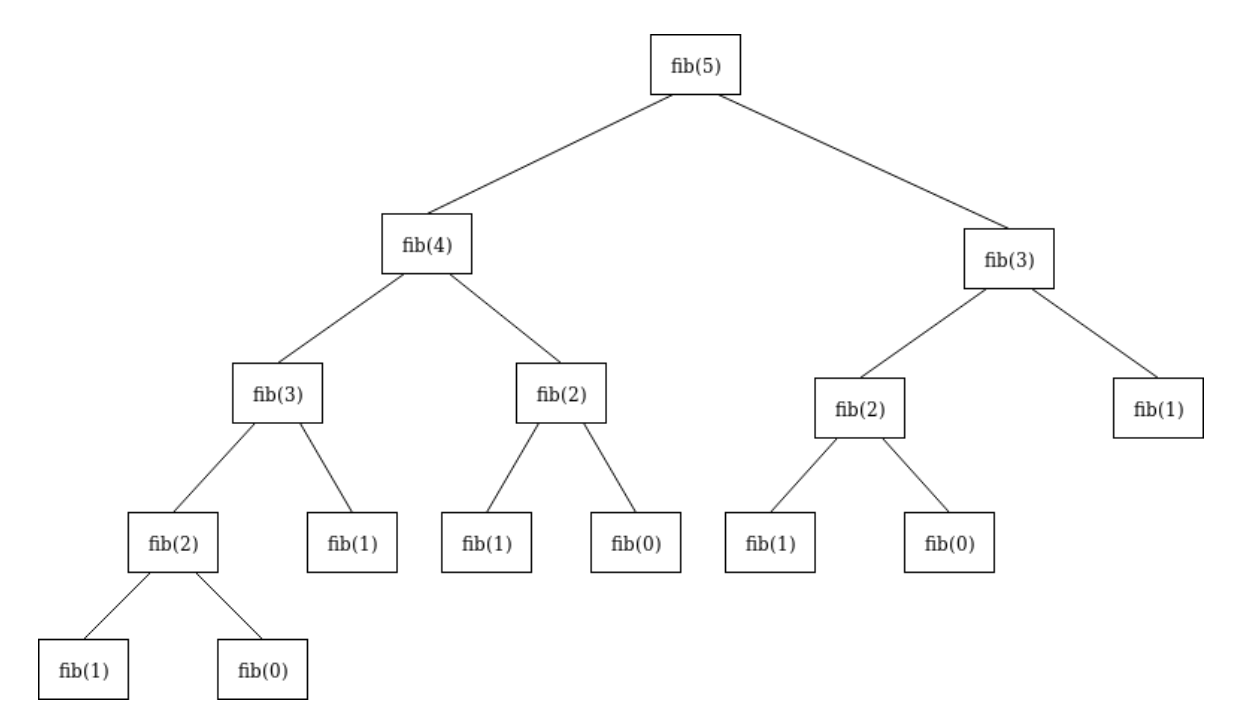# 509. 斐波那契数

``````class Solution {

public int fib(int N) {
if(N<=1){
return N;
}
return fib(N-1)+fib(N-2);
}

}
````````````class Solution {

public int fib(int N) {
if(N<=1){
return N;
}
int[] num = new int[N + 1];
num = 0;
num = 1;
for (int i=2; i <= N; i++) {
num[i] = num[i - 1] + num[i - 2];
}
return num[N];
}

}
``````

``````class Solution {

private static int[] cache = new int[]{0, 1};

public int fib(int N) {
int i = cache.length;
if (N >= i) {
cache = Arrays.copyOf(cache, N + 1);
for (; i <= N; i++) {
cache[i] = cache[i - 1] + cache[i - 2];
}
}
return cache[N];
}

}
``````